# HC Verma Solutions Class 12 Chapter 17 Alternating Current

HC Verma Solutions Class 12 Alternating current Chapter 17 are a great study tool to master Physics concepts given in this unit. The solutions for this chapter contain accurate and descriptive answers for all the problem questions present in the book. With the solution, students will be able to understand the different types of questions and also learn the right way to solve them. All in all, by using the solutions students can easily gain proficiency in understanding the key concepts along with developing better analytical skills.

With the help of HC Verma solutions, students who are planning to appear for competitive exams like JEE can also prepare well and be ready to tackle questions that could be asked from this unit. These solutions are the premier study materials for science students and for those who want to score better grades in the exams.

## Alternating Current Topics To Focus On

Students will need to learn about key topics like;

• AC Generator or AC Dynamo
• Instantaneous and RMS Current
• Simple AC Currents
• Vector Method
• AC Circuits
• Choke Coil
• Hot Wire Instruments
• DC Dynamo and Motor
• Transformer

## Class 12 Important Chapters In Chapter 17

1. When an AC source is connected to a capacitor what changes will occur with the rms current. Will it decrease or increase or will it remain constant when a dielectric slab is inserted into the capacitor?

2. If we take an LCR circuit do you think that the peak voltage across the inductor will be greater than the peak voltage of the source?

3. What happens when the voltage is stepped up in a transformer? Will there be any energy produced?

4. In a 220 volt AC source, what will the peak voltage read as?

(a) 440 V (b) 310 V (c) 160 V (d) 220 V

5. Can you tell where are transformers normally used in?

(a) AC circuits (b) DC circuits (c) in both AC and DC circuits (d) nowhere

## HC Verma Solutions Vol 2 Alternating Current- Chapter 17

Question 1: Find the time required for a 50 Hz alternating current to change its value from zero to the rms value.

Solution:

Current at any time = i = i0 sin (2πft)

Where,

i = Current at any time t.

i0 = Maximum value of the current in the circuit, and

f = Frequency of the alternating current

Here, irms = i0/√2

=> i0 sin (2πft) = i0/√2

or sin (2πft) = 1/√2 = sin(π/4)

=> 2πft = π/4

=> 2ft = 1/4

=> 2 x 50 x t = 1/4

=> t = 1/400 = 2.5 x 10-3 s

Question 2: The household supply of electricity is at 220 V (rms value) and 50 Hz. Find the peak voltage and the least possible time in which the voltage can change from the rms value to zero.

Solution:

Peak voltage = E0 = √2 x Erms

=> E0 = √2 x 220

=> E0 = 1.414 x 220

=> E0 = 311 V (approx)

Let us find the, time taken to reach the zero value from rms:

irms = i0/√2 = i0 sin ωt

or ωt = π/4

=> t = π/[4×2πf] [use, angular velocity = ω = 2πf]

=> t = π/[8πx50] = 1/400 = 2.5 x 10-3 s

Question 3: A bulb rated 60W at 220V is connected across a household supply of alternating voltage of 220V. Calculate the maximum instantaneous current through the filament

Solution:

We know, P = V2/R

or R = V2/P = 2/60 = 806.67

The maximum instantaneous current through the filament:

io = ε0/R ….(1)

ε0 = Instantaneous voltage = √2 x 220 = 311.08

(1)=> i0 = 311.08/806.67 = 0.39 A

Question 4: An electric bulb is designed to operate at 12 volts DC. If this bulb is connected to an AC source and given normal brightness, what would be the peak voltage of the source?

Solution:

Given: Voltage = 12V.

The peak voltage under given condition will be

Peak voltage = E0 = √2 x E= √2 x 12 = 16.97 = 17 V (approx)

[Use √2 = 1.414]

Question 5: The peak power consumed by a resistive coil when connected to an AC source is 80W. Find the energy consumed by the coil in 100 seconds which is many times larger than the time period of the source.

Solution:

Peak Power = P0 = 80 W

Then, instantaneous power = Prms = P0/2 = 40 W

Energy consumed by the coil in t = 100 s = P x t = 40 x 100 = 4000 J

Question 6: The dielectric strength of air is 3.0 × 106 V/m. A parallel-plate air-capacitor has area 20 cm2 and plate separation 0.10 mm. Find the maximum rms voltage of an AC source which can be safely connected to this capacitor.

Solution:

Dielectric strength of air = E = 3.0 × 106 V/m

Separation width = d = 0.10 mm and

Area = A = 20 cm2

Now,

Potential difference across the capacitor = V = E x d

= 3 x 106 x 1 x 10-4

= 300 V

The maximum rms voltage of an AC source which can be safely connected to this capacitor = Vrms = V/√2

= 300/√2

=> Vrms = 212 V

Question 7: The current in a discharging LR circuit is given by i = i0 e(–t/τ) where τ is the time constant of the circuit. Calculate the rms current for the period t = 0 to t = τ.

Solution:

The current in a discharging LR circuit i = i0 e(–t/τ)

So, the rms current for the period t = 0 to t = T :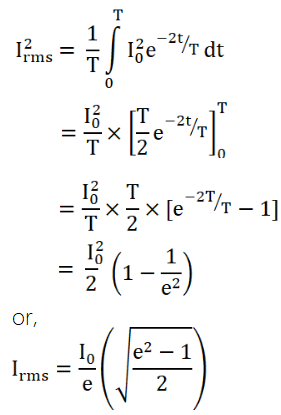Question 8: A capacitor of capacitance 10 μF is connected to an oscillator giving an output voltage ϵ = (10V) sin ωt. Find the peak currents in the circuit for ω = 10 s–1, 100 s–1, 500 s–1, 1000 s–1.

Solution:

Capacitance of the capacitor = C = 10 μF

Output voltage of the oscillator = ϵ = (10V) sin ωt

On comparing the output voltage of the oscillator with, ϵ = ϵ0

Peak voltage = ϵ0 = 10V

For capacitive circuit, peak current is i0 = ϵ0/xc

Where xc = 1/ωc

[ω = angular frequency and c = capacitance of capacitor]

At ω = 10 s-1

xc = 1/ωc = 1/[10 x 10-5]

ϵ0 = 10

=> i0 = ϵ0/xc = 10-3 A

At ω = 100 s-1

xc = 1/ωc = 1/[100 x 10-5]

ϵ0 = 10

=> i0 = ϵ0/xc = 10-2 A

At ω = 500 s-1

xc = 1/ωc = 1/[500 x 10-5]

ϵ0 = 10

=> i0 = ϵ0/xc = 5 x 10-2 A

At ω = 1000 s-1

xc = 1/ωc = 1/[1000x 10-5]

ϵ0 = 10

=> i0 = ϵ0/xc = 10-1 A

Question 9: A coil of inductance 5.0 mH and negligible resistance is connected to the oscillator of the previous problem. Find the peak currents in the circuit for ω= 100 s–1, 500 s–1, 1000s–1.

Solution:

Inductance of a coil = 5.0 mH and Peak voltage = ϵ0 =10 V

Let XL be the reactance of a coil and io be the peak current.

At ω = 100 s-1

XL = ωc = 0.005 x 100 = 0.5 ohm

=> i0 = ϵ0/xL = 20 A

At ω = 500 s-1

XL = ωc = 0.005 x 500 = 2.5 ohm

=> i0 = ϵ0/xL = 4 A

At ω = 1000 s-1

XL = ωc = 0.005 x 1000 = 5 ohm

=> i0 = ϵ0/xL = 2 A

Question 10: A coil has a resistance of 10 Ω and an inductance of 0.4 Henry. It is connected to an AC source of 6.5 V, 30/π Hz. Find the average power consumed in the circuit.

Solution:

Resistance= R = 10 Ω

Inductance = L = 0.4 Henry

Frequency = f = 30/π Hz

It is connected to an AC source (E) of 6.5 V

Impedance of a coil = Z = √[R2+XL2]

= √[R2+(2πfL)2]

rms current = irms = 6.5/Z and cos φ = R/Z

Average power consumed in the circuit:

Power = Vrms irms cosφ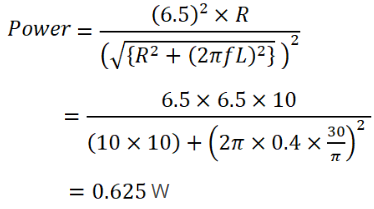Question 11: A resistor of resistance 100 Ω is connected to an AC source ϵ = (12 V) sin (250 π s–1) t. Find the energy dissipated as heat during t = 0 to t = 1.0 ms.

Solution:

Energy dissipated (E) as heat during t = 0 to t = 1.0 ms = 10-3 s: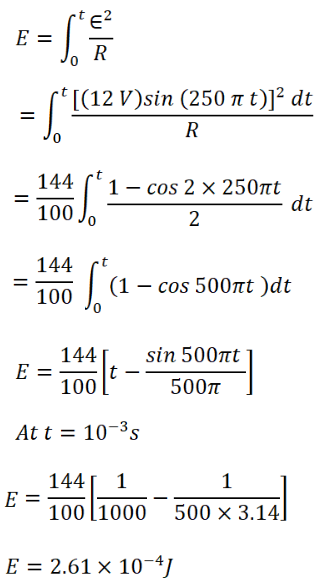[Using sin2A = (1-cos2A)/2 ]

Question 12: In a series RC circuit with an AC source R = 300Ω, C = 25 μF, ϵ0 = 50 V and v = 50/π Hz. Find the peak current and the average power dissipated in the circuit.

Solution:

Resistance = R = 300Ω,

Capacitance = C = 25 μF = 25 x 10-6 F

Frequency = v = 50/π Hz

Rms voltage = ϵ0 = 50/π V

We know, Reactance = XC = 1/ωC

= 1/2πvc

= 1/[2π x 25 x 10-6 x 50/π]

= 104/25

Now,

Impedance, Z

We know, Z2 = R2 + XC2

Z2 = (300)2 + (104/25)2

Z2 = 250000

Or Z = 500

Peak Current, io = ε0/Z = 50/500 = 0.1 A

Average power dissipated:

Power = Vrms i rms cos φ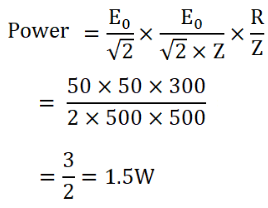Question 13: An electric bulb is designed to consume 55 W when operated at 110 volts. It is connected to a 220 V, 50 Hz line through a choke coil in series. What should be the inductance of the coil for which the bulb gets correct voltage?

Solution:

Power = P = 55W,

Bulb operated at voltage =V = 110V,

Voltage supplied =E = 220V

Frequency = f = 50Hz

Let “L” is the inductance of the coil for which the bulb gets correct voltage.

We know Resistance = R = V2/P = (110) 2/55 = 220 ohm

Angular velocity = ω = 2πf = 2π(50) = 100 π

Current in the circuit = i = E/Z = E/√[R2+(ωL)2]

Voltage drop across the resistor = V = iR = ER/√[R2+(ωL)2]

=> 110 = [220×220]/√[(220)2 +(100πL)2]

=> (220) 2 + (100πL) 2 = (440) 2

=> L2 = 1.4726

or L = 1.213 Hz

Question 14: In a series LCR circuit with an AC source, R = 300Ω, C = 20 μF, L = 1.0 Henry, ϵrms = 50 V and v = 50/π Hz. Find

(a) The rms current in the circuit and

(b) The rms potential differences across the capacitor, the resistor and the inductor. Note that the sum of the rms potential differences across the three elements is greater than the rms voltage of the source.

Solution:

Given:

R = 300Ω, C = 20 μF, L = 1.0 Henry, ϵrms = 50 V and v = 50/π Hz.

(a) rms current in the circuit:

Irms = ε/Z

Where Z is impedance in the circuit

We know, Z2 = R2 + (XC – XL) 2 …(1)

Given R = 300Ω

XC = 1/(2 πfC) = 1/(2 π x 50/ π x 20 x 10-6) = 104/20

XL = 2 πfL = 2 π x 50/ π x 1 = 100

(1)=> Z2 = (300)2 + (104/20 – 100) 2

Or Z2 = 250000

Or Z = 500

Thus, Io = 50/500 = 0.1 A

(b) Potential difference across the capacitor

We know VC = Io XC = 0.1 x 500 = 50 V

Potential difference across the resistor

VR = Io x R = 0.1 x 300 = 30 V

Potential difference across the inductor

VL = Io XL = 0.1 x 100 = 10 V

Now, Net potential drops = VC + VR + VL = 50+30+10 = 90 V

Rms Voltage = εrms = 50 V

=> Sum of all potential drops more than rms potential applied.

Question 15: Consider the situation of the previous problem. Find the average electric field energy stored in the capacitor and the average magnetic field energy stored in the coil.

Solution:

Resistance = R = 300Ω

Capacitance of capacitor =C = 20 μF

Frequency v = 50/π Hz

Inductance of inductor =L = 1.0 Henry

Voltage across the circuit ϵrms = 50 V

Current = I0 = 0.1 A [From previous problem]

Electric energy stored in capacitor: E_C = (1/2) CV2

= (1/2) x 20 x 10-6 x 50 x 50

= 25 x 10-3 J

= 25 mJ

Magnetic field energy stored in the coil: E_M = (1/2) LI02

= (1/2) x 1 x (0.1) 2 x 5 x 10-3

= 5 x 10-3 J

= 5 mJ

Question 16: An inductance of 2.0 H, a capacitance of 18 μF and a resistance of 10 kΩ are connected to an AC source of 20 V with adjustable frequency.

(a) What frequency should be chosen to maximize the current in the circuit?

(b) What is the value of this maximum current?

Solution:

(a) For current to be maximum in a circuit: XC = XL

Where, XC and XL resonance across capacitor and inductor respectively.

1/ωC = ωL

=> ω2 = 1/LC

=> ω2 = 1/[2x18x10-6]

=> ω2 = 106/36

=> ω = 103/6

Also ω = 2πf

So, f = 1000/[6×2π] = 27 Hz

(b) Max. Current = I = E/R

=> I = 20/(10×103) = 2 mA

Question 17: An inductor-coil, a capacitor and an AC source of rms voltage 24 V are connected in series. When the frequency of the source is varied, a maximum rms current of 6.0 A is observed. If this inductor coil is connected to a battery of emf 12 V and internal resistance 4.0Ω, what will be the current?

Solution:

rms voltage = Erms = 24 V

Internal resistance = r = 4 ohm

rms current = Irms = 6A

rms resistance = R = Erms/Irms = 24/6 = 4 ohm

If this inductor coil is connected to a battery of emf 12 V and internal resistance 4.0Ω, say R’ = 4.0Ω, the steady current

I = E/R’

Net resistance = R’ = R + r’ = 4 + 4 = 8 ohm

The steady current = I = 12/8 = 1.5 A

Question 18: Figure shows a typical circuit for low-pass filter. An AC input Vi = 10 mV is applied at the left end and the output V0 is received at the right end. Find the output voltages for v = 10 kHz, 100 k Hz, 1.0 MHz and 10.0 MHz Note that as the frequency is increased the output decreases and hence the name low-pass filter.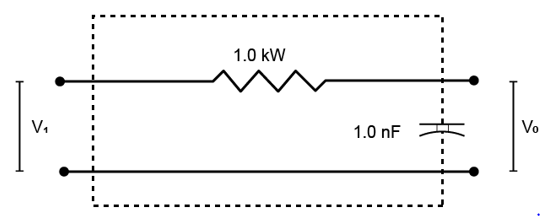Solution:

Voltage = V = 10 × 10-3 V

Resistance = R = 1 × 103 ohm

Capacitance = C = 10 × 10-9 F

At frequency, f = 10 kHz

Reactance = XC = 1/ωC

Where ω = angular velocity = 2πf

Here, ωC = 2πfC = 2π x 10 x 103 x 10 x 10-9 = 104/2π

=> XC = 5000/π

Again, Impedance = Z = √[R2+XC2]

Here, R2 + XC2 = (1×103) 2 + (5000/π)2

R2 + XC2 = 106 + (5000/π)2

=> Z = √[106 + (5000/π)2]

Current = Io = V/Z

Io = [10 × 10-3]/ √[106 + (5000/π)2]

Now, Output voltage = V0 = I0 XC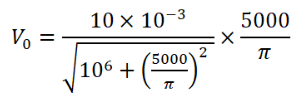Vo = 16.12 V = 16.1 mV

At frequency, f = 100 kHz

Reactance = XC = 1/ωC

Where ω = angular velocity = 2πf

XC = 1/2πfC = 1/[2π x 100 x 103 x 10 x 10-9 ] = 103/2π

=> XC = 500/π

Again, Impedance = Z = √[R2+XC2]

Here, R2 + XC2 = (1×103) 2 + (500/π)2

R2 + XC2 = 106 + (500/π)2

=> Z = √[106 + (500/π)2]

Current = Io = V/Z

Io = [10 × 10-3]/ √[106 + (500/π)2]

Now, Output voltage = V0 = I0 XC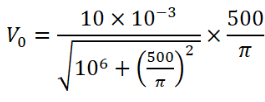Vo = 1.612 V = 1.6 mV

At frequency, f = 1 MHz

Reactance = XC = 1/ωC

Where ω = angular velocity = 2πf

XC = 1/2πfC = 1/[2π x 106 x 10 x 10-9 ] = 102/2π

=> XC = 50/π

Again, Impedance = Z = √[R2+XC2]

Here, R2 + XC2 = (1×103) 2 + (50/π)2

R2 + XC2 = 106 + (50/π)2

=> Z = √[106 + (50/π)2]

Current = Io = V/Z

Io = [10 × 10-3]/ √[106 + (50/π)2]

Now, Output voltage = V0 = I0 XC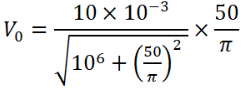Vo = 0.1612 V = 0.16 mV

At frequency, f = 10 MHz

Reactance = XC = 1/ωC

Where ω = angular velocity = 2πf

XC = 1/2πfC = 1/[2π x 107 x 10 x 10-9 ] = 10/2π

=> XC = 5/π

Again, Impedance = Z = √[R2+XC2]

Here, R2 + XC2 = (1×103) 2 + (5/π)2

R2 + XC2 = 106 + (5/π)2

=> Z = √[106 + (5/π)2]

Current = Io = V/Z

Io = [10 × 10-3]/ √[106 + (5/π)2]

Now, Output voltage = V0 = I0 XC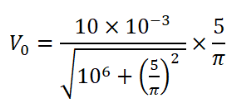Vo = 0.01612 V = 16 μV

Question 19: A transformer has 50 turns in the primary and 100 in the secondary. If the primary is connected to a 220 V DC supply, what will be the voltage across the secondary?

Solution:

If the primary is connected to a 220 V DC supply, the voltage across the secondary is zero.

Reason: A transformer works on the principle of electromagnetic induction, which is only possible on AC.

The induced current in the secondary coil is zero. Thus, output voltage will be zero.